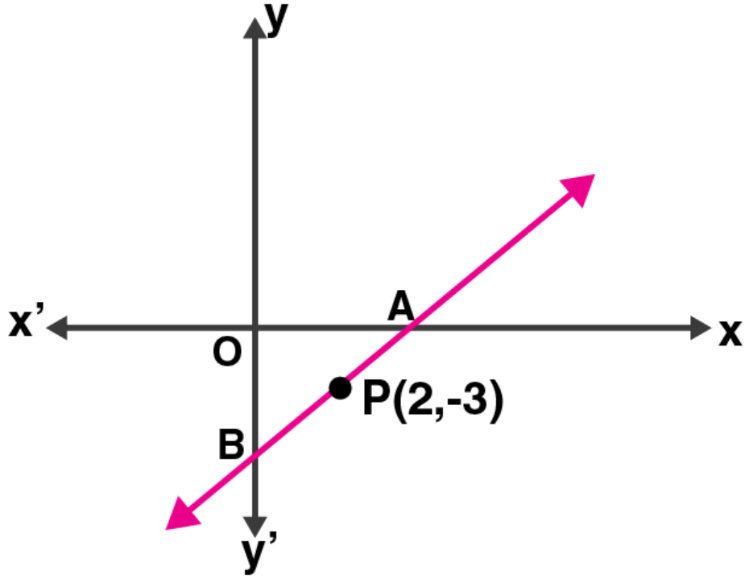Newbie

# A and B are two points on the x-axis and y-axis respectively. P (2, – 3) is the mid point of AB. Find the (i) the co-ordinates of A and B. (ii) the slope of the line AB. (iii) the equation of the line AB.

• 0

M.L Aggarwal book Important Question of class 10 chapter Based on Equation of a Straight Line for ICSE BOARD.
Two points of x and y axis is given
You have to find the co-ordinates ,slope of line ,equation of the line asked in question
This is the Question Number 30, Exercise 12.1 of M.L Aggarwal.

Share

1.Given, points A and B are on x-axis and y-axis respectively

Let co-ordinates of A be (x, 0) and of B be (0, y)

And P (2, -3) is the midpoint of AB

So, we have

2 = (x + 0)/2 and -3 = (0 + y)/2

x = 4 and y = -6

(i) Hence, the co-ordinates of A are (4, 0) and of B are (0, -6).

(ii) Slope of AB = y2 – y1/ x2 – x1

= (-6 – 0)/ (0 – 4)

= -6/-4 = 3/2 = m

(iii) Equation of AB will be

y – y1 = m (x – x1)

y – (-3) = 3/2 (x – 2) [As P lies on it]

y + 3 = 3/2 (x – 2)

2y + 6 = 3x – 6

3x – 2y – 12 = 0

• 0